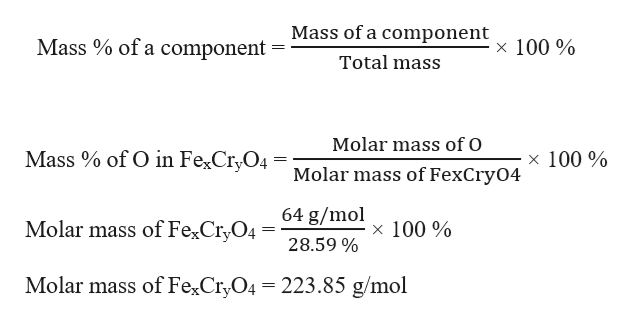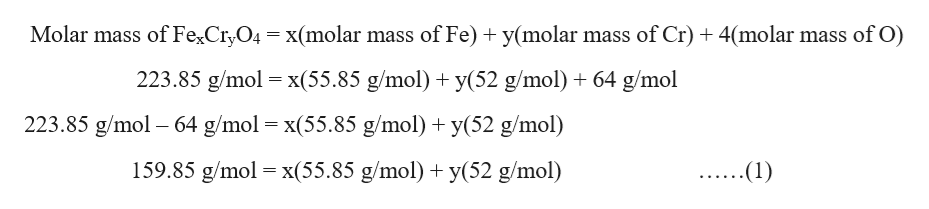A chromium containing compound has a formula FexCryO4 and is 28.59% oxygen by mass Find x and y

Question

A chromium containing compound has a formula FexCryO4 and is 28.59% oxygen by mass

Find x and y

Step 1

Given, mass percentage of O in FexCryO4 = 28.59%

Molar mass of 4 O in FexCryO4 = 4 × 16 g/mol = 64 g/mol

Mass percentage is expressed as:help_outlineImage TranscriptioncloseMass of a component Mass % of a component 100 % Total mass Molar mass of O of O in Fe2Cr,O4 = Molar mass of FexCry04 Mass x 100% 64 g/mol Molar mass of FexCryO4 x 100% 28.59 % Molar mass of FexCryO4 223.85 g/mol fullscreen
Step 2

The molar mass of FexCryO4 can be expressed as:help_outlineImage TranscriptioncloseMolar mass of FexCryO4 x(molar mass of Fe) y(molar mass of Cr) 4(molar mass of O) 223.85 g/mol x(55.85 g/mol) y(52 g/mol) 64 g/mol 223.85 g/mol 64 g/mol x(55.85 g/mol)y(52 g/mol) = 159.85 g/mol x(55.85 g/mol) y(52 g/mol) (1) fullscreen
Step 3

So, the sum of [x(55.85 g/mol) + y(52 g/mol)] must not exceed 159.85 g/mol. And there must be at least one Fe and one Cr in FexCryO4. Now, from equation (1) we ...

Want to see the full answer?

See Solution

Want to see this answer and more?

Our solutions are written by experts, many with advanced degrees, and available 24/7

See Solution
Tagged in

General Chemistry